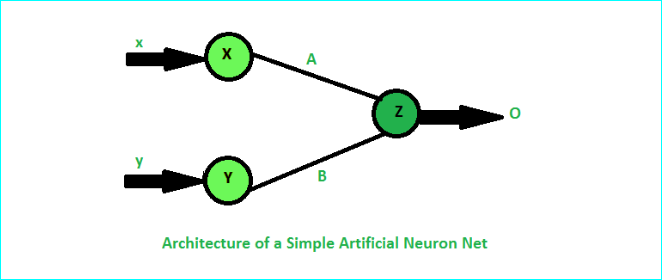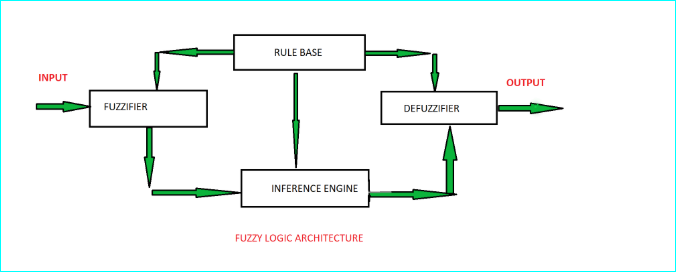Difference between Neural Network And Fuzzy Logic

• Last Updated : 17 Jul, 2020

Neural Network:
Neural network is an information processing system that is inspired by the way biological nervous systems such as brain process information. A neural network is composed of a large number of interconnected processing elements known as neurons which are used to solve problems. A neural network is an attempt to make a computer model of the human brain and neural networks are parallel computing devices. The simple diagram of the neural network is as shown below:Fuzzy Logic:
The term fuzzy represents the things which are not clear. In the real world many times we find a situation where we can’t determine whether the state is true or false, their fuzzy logic provides very valuable flexibility for reasoning. In this way, we can consider the inaccuracies and uncertainties of any situation. The simple diagram of fuzzy logic is as shown below:Difference between Neural Network And Fuzzy Logic

Neural NetworkFuzzy Logic
This system can not easily modified.This system can easily modified.
It trains itself by learning from data setEverything must be defined explicitly.
It is complex than fuzzy logic.It is simpler than neural network.
It helps to perform predictions.It helps to perform pattern recognition.
Difficult to extract knowledge.Knowledge can easily extracted.
It based on learning.It doesn’t base on learning.

Attention reader! Don’t stop learning now. Get hold of all the important Machine Learning Concepts with the Machine Learning Foundation Course at a student-friendly price and become industry ready.

My Personal Notes arrow_drop_up